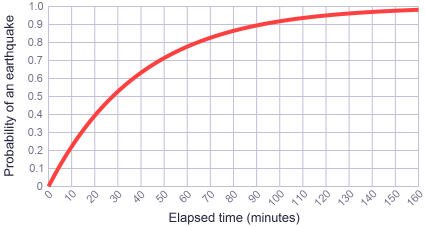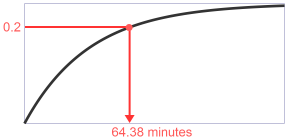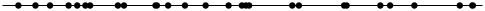# How to Generate Random Timings for a Poisson Process

What’s a Poisson process, and how is it useful?

Any time you have events which occur individually at random moments, but which tend to occur at an average rate when viewed as a group, you have a Poisson process.

For example, the USGS estimates that each year, there are approximately 13000 earthquakes of magnitude 4+ around the world. Those earthquakes are scattered randomly throughout the year, but there are more or less 13000 per year. That’s one example of a Poisson process. The Wikipedia page lists several others.

In statistics, there are a bunch of functions and equations to help model a Poisson process. I’ll present one of those functions in this post, and demonstrate its use in writing a simulation.

## The Exponential Distribution

If 13000 such earthquakes happen every year, it means that, on average, one earthquake happens every 40 minutes. So, let’s define a variable λ = $$\frac{1}{40}$$ and call it the rate parameter. The rate parameter λ is a measure of frequency: the average rate of events (in this case, earthquakes) per unit of time (in this case, minutes).

Knowing this, we can ask questions like, what is the probability that an earthquake will happen within the next minute? What’s the probability within the next 10 minutes? There’s a well-known function to answer such questions. It’s called the cumulative distribution function for the exponential distribution, and it looks like this:

$$F(x) = 1 - e^{-\lambda x}$$Basically, the more time passes, the more likely it is that, somewhere in the world, an earthquake will occur. The word “exponential”, in this context, actually refers to exponential decay. As time passes, the probability of having no earthquake decays towards zero – and correspondingly, the probability of having at least one earthquake increases towards one.

Plugging in a few values, we find that:

• The probability of having an earthquake within the next minute is $$F(1) \approx 0.0247$$. This value is pretty close to $$\frac{1}{40}$$, our prescribed earthquake frequency, but it’s not equal.
• The probability of having an earthquake within the next 10 minutes is $$F(10) \approx 0.221$$.

In particular, note that after 40 minutes – the prescribed average time between earthquakes – the probability is only $$F(40) \approx 0.632$$. So, given any 40 minute interval of time, it’s pretty likely that we’ll have an earthquake within that time interval, but it won’t always happen.

## Writing a Simulation

Now, suppose we want to simulate the occurrence of earthquakes in a game engine, or some other kind of program. First, we need to figure out when each earthquake should begin.

One approach is to loop, and after each interval of X minutes, sample a random floating-point value between 0 and 1. If this number is less than $$F(X)$$, then start an earthquake! X could even be a fractional value, so you could sample several times per minute, or even several times per second. This approach will probably work just fine, as long as your random number generator is uniform and offers enough numerical precision. However, if you intend to sample 60 times per second, with λ = $$\frac{1}{40}$$, you’ll need at least 18 bits of precision from the random number generator, which the Standard C Runtime Library doesn’t always offer.

Another approach is to sidestep the whole sampling strategy, and simply write a function to determine the exact amount of time until the next earthquake. This function should return random numbers, but not the uniform kind of random number produced by most generators. We want to generate random numbers in a way that follows our exponential distribution.

Donald Knuth describes a way to generate such values in §3.4.1 (D) of The Art of Computer Programming. Simply choose a random point on the y-axis between 0 and 1, distributed uniformly, and locate the corresponding time value on the x-axis. For example, if we choose the point 0.2 from the top of the graph, the time until our next earthquake would be 64.38 minutes.Given that the inverse of the exponential function is ln, it’s pretty easy to write this analytically, where U is the random value between 0 and 1:

## The Implementation

Here’s one way to implement it in Python. Note that you can’t pass zero to math.log, but we avoid that by subtracting the result of random.random, which is always less than one, from one.

import math
import random

def nextTime(rateParameter):
return -math.log(1.0 - random.random()) / rateParameter


Update: After writing this post, I learned that Python has a standard library function which does exactly the same thing as nextTime. It’s called random.expovariate.

Here are a few sample calls. The values look pretty reasonable:

>>> nextTime(1/40.0)
91.074923814190498
>>> nextTime(1/40.0)
46.88573030224817
>>> nextTime(1/40.0)
14.965086245136733
>>> nextTime(1/40.0)
26.902965535881194


Let’s run some tests to make sure that the average time returned by this function really is 40. The following expression calculates the average of one million calls, and the results are pretty consistent. I’m always amazed to see randomness behaving the way we want!

>>> sum([nextTime(1/40.0) for i in xrange(1000000)]) / 1000000
39.985564565743751
>>> sum([nextTime(1/40.0) for i in xrange(1000000)]) / 1000000
40.029018385760551
>>> sum([nextTime(1/40.0) for i in xrange(1000000)]) / 1000000
40.016843319423266
>>> sum([nextTime(1/40.0) for i in xrange(1000000)]) / 1000000
39.965097296560664


Just for fun, here’s a series of points spaced according to the output of nextTime. This is basically what a Poisson process looks like when plotted along a timeline:And here’s an implementation of nextTime in C, using the standard library’s random number generator. Again, we’re careful not to pass zero to logf.

#include <math.h>
#include <stdlib.h>

float nextTime(float rateParameter)
{
return -logf(1.0f - (float) random() / (RAND_MAX + 1)) / rateParameter;
}


This technique could have various applications in a game engine, such as spawning particles from a particle emitter, or choosing moments when an AI could take a decision. I also use it in my next post, to measure the performance of threads which hold a lock for various intervals of time.

Any stats experts out there? If I’ve abused any terminology, or if you see any way to improve this post, I’d be interested in your comments.